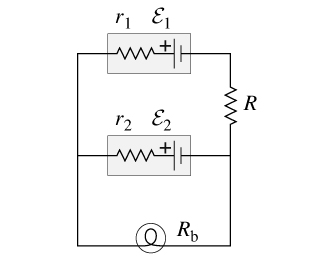# Problem: The circuit diagram below shows two emf sources and a bulb connected in parallel. Also connected in the circuit is a resistor with resistance R = 0.2Ω. The resistance of the bulb is Rb = 0.5 Ω , and each of the sources has internal resistance: r1 = 0.025 Ω and r2 = 0.02 Ω.If ε1 = 13.0 V and ε2 = 5.0 V , Part A. Calculate the current I2 flowing in emf source ε2Part BCalculate the current I1 flowing in emf source ε1.Express your answer in amperes to three significant figures.Part CA useful strategy to evaluate your answer is to consider a loop other than the ones you used to solve the problem; if the sum of potential drops around this loop isn’t zero, you made an error somewhere in your calculations. Begin by calculating the current I1 flowing through the emf source ε1.Express your answer in amperes to three significant figures.

###### FREE Expert Solution

By Kirchhoff's junction rule:

I = I1 - I2

By Kirchhoff's loop rule:

In the upper loop

$\begin{array}{rcl}{\mathbf{\epsilon }}_{\mathbf{1}}\mathbf{-}{\mathbf{I}}_{\mathbf{1}}{\mathbf{r}}_{\mathbf{1}}\mathbf{-}{\mathbf{I}}_{\mathbf{2}}{\mathbf{r}}_{\mathbf{2}}\mathbf{-}{\mathbf{\epsilon }}_{\mathbf{2}}\mathbf{-}{\mathbf{I}}_{\mathbf{1}}\mathbf{R}& \mathbf{=}& \mathbf{0}\\ \mathbf{13}\mathbf{.}\mathbf{0}\mathbf{-}\mathbf{0}\mathbf{.}\mathbf{025}{\mathbf{I}}_{\mathbf{1}}\mathbf{-}\mathbf{0}\mathbf{.}\mathbf{02}{\mathbf{I}}_{\mathbf{2}}\mathbf{-}\mathbf{5}\mathbf{.}\mathbf{0}\mathbf{-}\mathbf{0}\mathbf{.}\mathbf{2}{\mathbf{I}}_{\mathbf{1}}& \mathbf{=}& \mathbf{0}\end{array}$

8 = 0.225I1 + 0.02I2

In the lower loop:

100% (337 ratings)###### Problem Details

The circuit diagram below shows two emf sources and a bulb connected in parallel. Also connected in the circuit is a resistor with resistance R = 0.2Ω. The resistance of the bulb is Rb = 0.5 Ω , and each of the sources has internal resistance: r1 = 0.025 Ω and r2 = 0.02 Ω.

If ε1 = 13.0 V and ε2 = 5.0 V ,

Part A. Calculate the current I2 flowing in emf source ε2Part B

Calculate the current I1 flowing in emf source ε1.

Part C

A useful strategy to evaluate your answer is to consider a loop other than the ones you used to solve the problem; if the sum of potential drops around this loop isn’t zero, you made an error somewhere in your calculations. Begin by calculating the current I1 flowing through the emf source ε1.